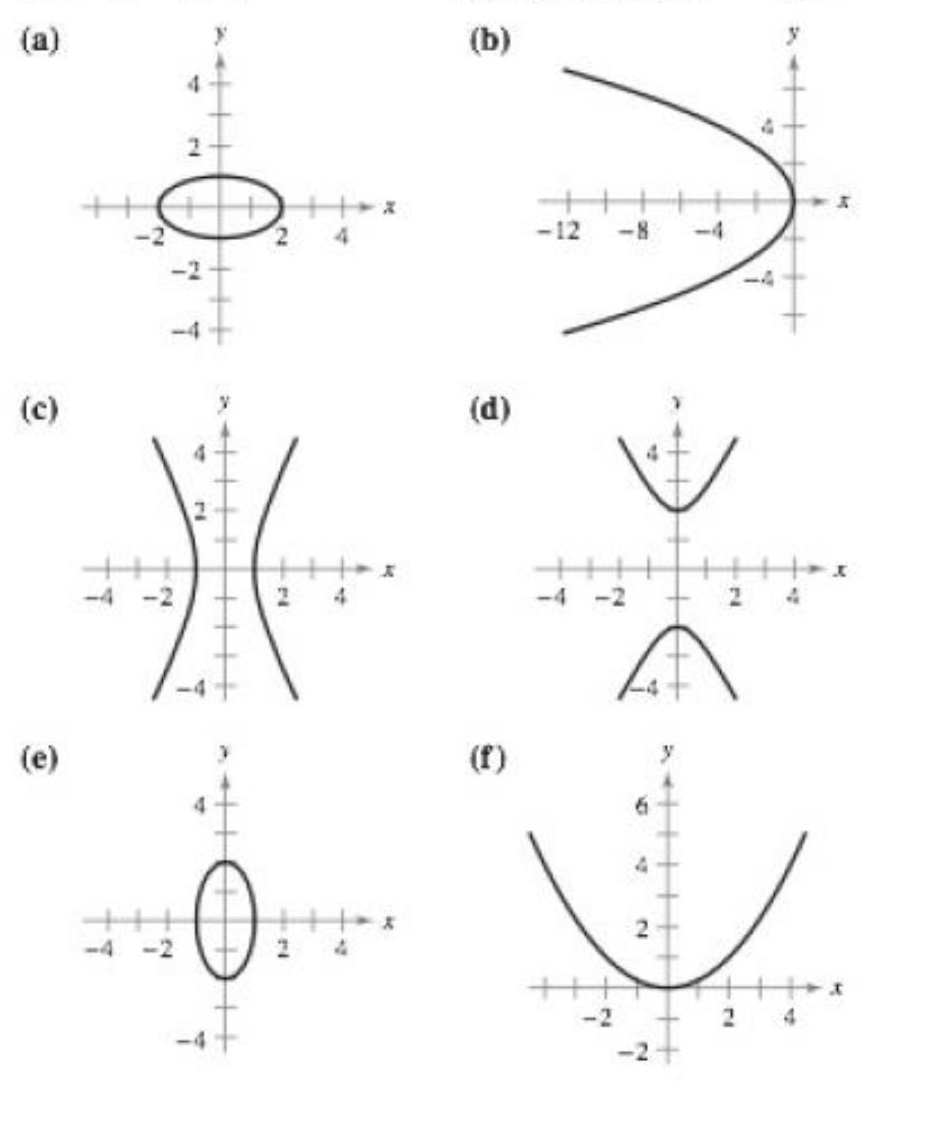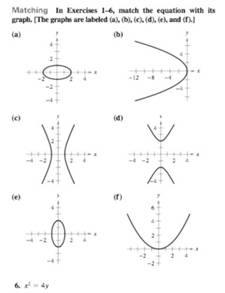Chapter 10, Problem 5RE

Chapter
Section
Textbook Problem

# Matching In Exercises 1-6, match the equation with its graph. [The graphs are labeled (a), (b), (c), (d), (e), and (f).]x 2   +   4 y 2   =   4

To determine

If the equation x2+4y2=4 matches with its graph when the options are:Explanation

Given:

The options are:

Here, x and y both are squared terms and both of their coefficients are positive. Thus, this equation represents an ellipse.

Now, in order to find whether the ellipse is horizontal or vertical, represent the given equation in the standard form of an ellipse.

The given equation is:

x2+4y2=4

Divide both the sides by 4:

14x2+y2=1

Rewrite in standard form as:

x222+y212=1

This is of the form:

(xh)

### Still sussing out bartleby?

Check out a sample textbook solution.

See a sample solution

#### The Solution to Your Study Problems

Bartleby provides explanations to thousands of textbook problems written by our experts, many with advanced degrees!

Get Started

#### If f(x)=limtxsectsecxtx, find the value of f'(/4).

Single Variable Calculus: Early Transcendentals, Volume I

#### Can the vectors in Problems 1-4 be probability vectors? If not, why? 2.

Mathematical Applications for the Management, Life, and Social Sciences

#### limx(12)x2= a) 0 b) 1 c) d) does not exist

Study Guide for Stewart's Single Variable Calculus: Early Transcendentals, 8th

#### Find the domain of f(x)=2x3x2x2.

College Algebra (MindTap Course List)